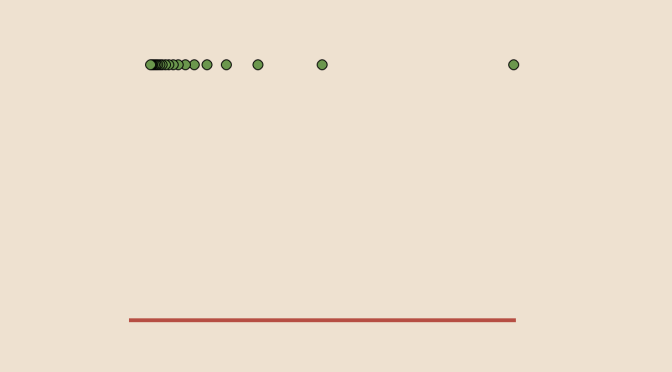Counterexamples on function limits (part 1)

Let $$f$$ and $$g$$ be two real functions and $$a \in \mathbb R \cup \{+\infty\}$$. We provide here examples and counterexamples regarding the limits of $$f$$ and $$g$$.

If $$f$$ has a limit as $$x$$ tends to $$a$$ then $$\vert f \vert$$ also?

This is true. It is a consequence of the reverse triangle inequality $\left\vert \vert f(x) \vert – \vert l \vert \right\vert \le \vert f(x) – l \vert$ Hence if $$\displaystyle \lim\limits_{x \to a} f(x) = l$$, $$\displaystyle \lim\limits_{x \to a} \vert f(x) \vert = \vert l \vert$$

Is the converse of previous statement also true?

It is not. Consider the function defined by: $\begin{array}{l|rcl} f : & \mathbb R & \longrightarrow & \mathbb R \\ & \frac{1}{n} & \longmapsto & -1 \text{ for } n \ge 1 \text{ integer} \\ & x & \longmapsto & 1 \text{ otherwise} \end{array}$ $$\vert f \vert$$ is the constant function equal to $$1$$, hence $$\vert f \vert$$ has $$1$$ for limit as $$x$$ tends to zero. However $$\lim\limits_{x \to 0} f(x)$$ doesn’t exist.

If $$f$$ has a limit at $$a$$ and $$g$$ not, then $$f+g$$ doesn’t have a limit at $$a$$?

This is a tricky question! The case where $$\displaystyle \lim\limits_{x \to a} f(x) = l$$ is finite is covered in the next paragraph. If $$l$$ is infinite, $$f+g$$ can have a limit at $$a$$. Consider $\begin{array}{l|rcl} g : & (0,1) & \longrightarrow & \mathbb R \\ & x & \longmapsto & \sin \frac{1}{x} \end{array}$ and $\begin{array}{l|rcl} f : & (0,1) & \longrightarrow & \mathbb R \\ & x & \longmapsto & \frac{1}{x} \end{array}$ We have $$\displaystyle \lim\limits_{x \to 0^+} f(x)=+\infty$$, $$g$$ doesn’t have a right limit at $$0$$ but $$\displaystyle \lim\limits_{x \to 0^+} (f+g)(x)=+\infty$$

If $$f$$ has a finite limit at $$a$$ and $$g$$ not, then $$f+g$$ doesn’t have a finite limit at $$a$$?

This is true. If $$f+g$$ was having a finite limit at $$a$$, writing $$g=(f+g)-f$$ we see that $$g$$ would have a limit as the difference of two functions having a limit at a point has a limit at that point. In contradiction with our hypothesis.

If $$f$$ is defined on (u,v) and monotonic, then $$f$$ has left and right limits at all $$a \in (u,v)$$?

This is true. Suppose that $$f$$ is increasing (if $$f$$ is decreasing, just consider $$-f$$). We prove that $$f$$ has a left limit for all $$a \in (u,v)$$, the proof being similar for the right limit. Consider the set $$S=\{f(x) | x < a\}$$. $$S$$ is bounded by $$f(a)$$ and not empty as $$f(\frac{u+a}{2}) \in S$$. Therefore $$S$$ has a least upper bound $$l$$. For any $$\epsilon > 0$$, there exists $$y < a$$ with $$l - \epsilon \le f(y) \le l$$. As $$f$$ is increasing we have for $$y < x < a$$ $f-\epsilon \le f(y) \le f(x) \le l$ proving that $$\displaystyle \lim\limits_{x \to a^-} f(x) = l$$.

If $$f$$ has finite left and right limits for all $$a \in [u,v]$$ then $$f$$ is bounded on $$[u,v]$$?

This is true. Suppose that $$(a_n)$$ is a sequence of $$[u,v]$$ with $$(f(a_n))$$ unbounded. As $$[u,v]$$ is compact one can find a converging subsequence $$(a_{\alpha_n})$$ converging to $$a \in [u,v]$$ with $$(f(a_{\alpha_n}))$$ unbounded. Then either an infinite number of elements of $$(a_{\alpha_n})$$ are less than $$a$$ and $$f$$ cannot have a finite left limit at $$a$$ or an infinite number of elements of $$(a_{\alpha_n})$$ are larger than $$a$$ and $$f$$ cannot have a finite right limit at $$a$$. In both cases we get a contradiction, hence $$f$$ is bounded on $$[u,v]$$.

What about the reverse of previous statement?

It is false. Consider the Dirichlet function $\begin{array}{l|rcl} f : & \mathbb R & \longrightarrow & \mathbb R \\ & x & \longmapsto & 1 \text{ for } x \in \mathbb Q \\ & x & \longmapsto & 0 \text{ for } x \in \mathbb R \setminus \mathbb Q \end{array}$ $$f$$ is bounded but doesn’t have a limit at any $$a \in \mathbb R$$ as $$\mathbb Q$$ is dense in $$[0,1]$$.

A real function $$f$$ has a limit at least one point $$a \in \mathbb R$$?

Is wrong. Consider again Dirichlet function of previous paragraph.

A function continuous at $$a$$ is continuous on an interval containing $$a$$?

Doesn’t hold. Consider $\begin{array}{l|rcl} f : & \mathbb R & \longrightarrow & \mathbb R \\ & x & \longmapsto & \vert x \vert \text{ for } x \in \mathbb Q \\ & x & \longmapsto & 0 \text{ for } x \in \mathbb R \setminus \mathbb Q \end{array}$ $$f$$ is continuous at $$0$$ as we have $$\vert f(x) \vert \le \vert x \vert$$ for all $$x \in \mathbb R$$.

However for $$a \neq 0$$ $$f$$ is not continuous at $$a$$ as:

• if $$a \in \mathbb Q$$, we have $$f(a) > 0$$ and $$f(x_n)=0$$ for a sequence $$(x_n)$$ of irrational points converging to $$a$$.
• if $$a \in \mathbb R \setminus \mathbb Q$$, we have $$f(a) = 0$$ and $$f(x_n) \to \vert a \vert\ > 0$$ for a sequence $$(x_n)$$ of rational points converging to $$a$$.

There exists at least an interval $$(u,v)$$ on which $$f$$ is bounded?

Is wrong. Have a look to a nowhere locally bounded function.